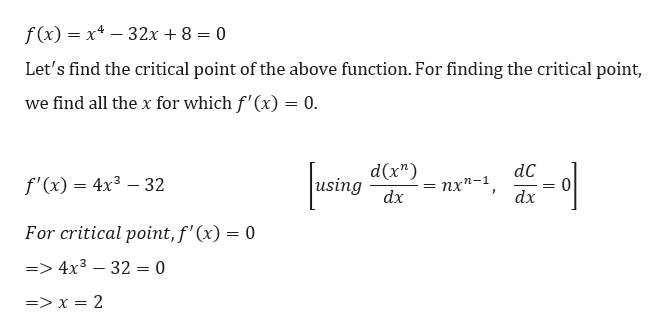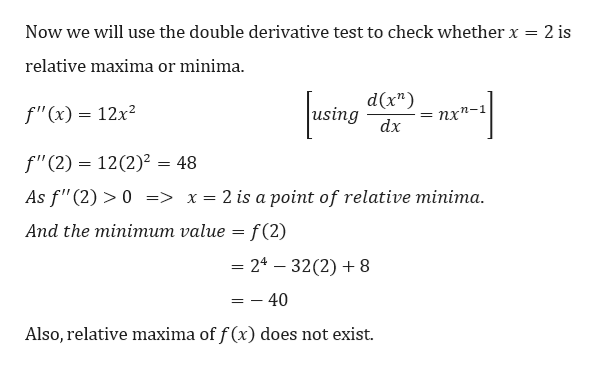Find all relative extrema of the function. (If an answer does not exist, enter DNE.)f(x) = x4 − 32x + 8relative maximum (x, y) = relative minimum (x, y) =

Question

Find all relative extrema of the function. (If an answer does not exist, enter DNE.)
f(x) = x4 − 32x + 8
relative maximum (x, y) =

relative minimum (x, y) =

Step 1

Given,help_outlineImage Transcriptionclosef(x) x4-32x + 8 = 0 Let's find the critical point of the above function. For finding the critical point, we find all the x for which f'(x) = 0. d(x") using dC f'(x) 4x3 32 пх^-1 dx dx For critical point, f'(x) = 0 =4x3 32 = 0 => x = 2 fullscreen
Step 2

Also,

...help_outlineImage TranscriptioncloseNow we will use the double derivative test to check whether x = 2 is relative maxima or minima d(x") using f"(x) = 12x2 пх*- dx f"(2) 12(2)2 = 48 As f"(2)>0 => x= 2 is a point of relative minima Аnd the miniтит value — f (2) 24-32(2)8 - 40 Also, relative maxima of f (x) does not exist fullscreen

Want to see the full answer?

See Solution

Want to see this answer and more?

Our solutions are written by experts, many with advanced degrees, and available 24/7

See Solution
Tagged in

Derivative